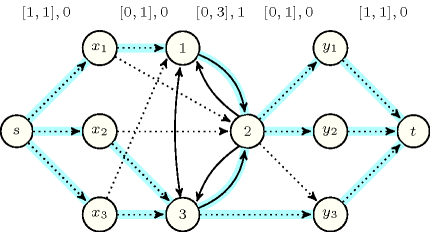### 3.7.153. Minimum cost flow

A constraint for which there is a filtering algorithm based on an algorithm that finds a minimum cost flow in a graph. This graph is usually constructed from the variables of the constraint as well as from their potential values. Figure 3.7.42 illustrates the minimum cost flow model used for the $\mathrm{𝚜𝚘𝚏𝚝}_\mathrm{𝚜𝚊𝚖𝚎}_\mathrm{𝚟𝚊𝚛}$ constraint. The demand and the capacity of the arcs are depicted by an interval on top of the corresponding arcs. The weight is given after that interval: a weight of 0 (respectively 1) is depicted by a dotted (respectively plain) arc. Weights of 1 are assigned to arcs linking two values since they model the correction of a discrepancy between variables ${x}_{1}$, ${x}_{2}$, ${x}_{3}$ and variables ${y}_{1}$, ${y}_{2}$, ${y}_{3}$. Blue arcs represent the feasible flow corresponding to the solution $\mathrm{𝚜𝚘𝚏𝚝}_\mathrm{𝚜𝚊𝚖𝚎}_\mathrm{𝚟𝚊𝚛}$$\left(2,〈1,3,3〉,〈2,2,3〉\right)$.

##### Figure 3.7.42. Minimum cost flow model for the $\mathrm{𝚜𝚘𝚏𝚝}_\mathrm{𝚜𝚊𝚖𝚎}_\mathrm{𝚟𝚊𝚛}$ constraint described in Table 3.7.42##### Table 3.7.42. Domains of the variables for the $\mathrm{𝚜𝚘𝚏𝚝}_\mathrm{𝚜𝚊𝚖𝚎}_\mathrm{𝚟𝚊𝚛}$ constraint of Figure 3.7.42.
$i$$\mathrm{𝑑𝑜𝑚}\left({x}_{i}\right)$$i$$\mathrm{𝑑𝑜𝑚}\left({y}_{i}\right)$
1$\left\{1,2\right\}$1$\left\{2\right\}$
2$\left\{2,3\right\}$2$\left\{2\right\}$
3$\left\{1,3\right\}$3$\left\{2,3\right\}$